Weston B.
University of Southern California

Biography

College student passionate about computer science, mathematics and physics.

Education

BA Interactive Entertainment
University of Southern California

Weston's Videos

01:50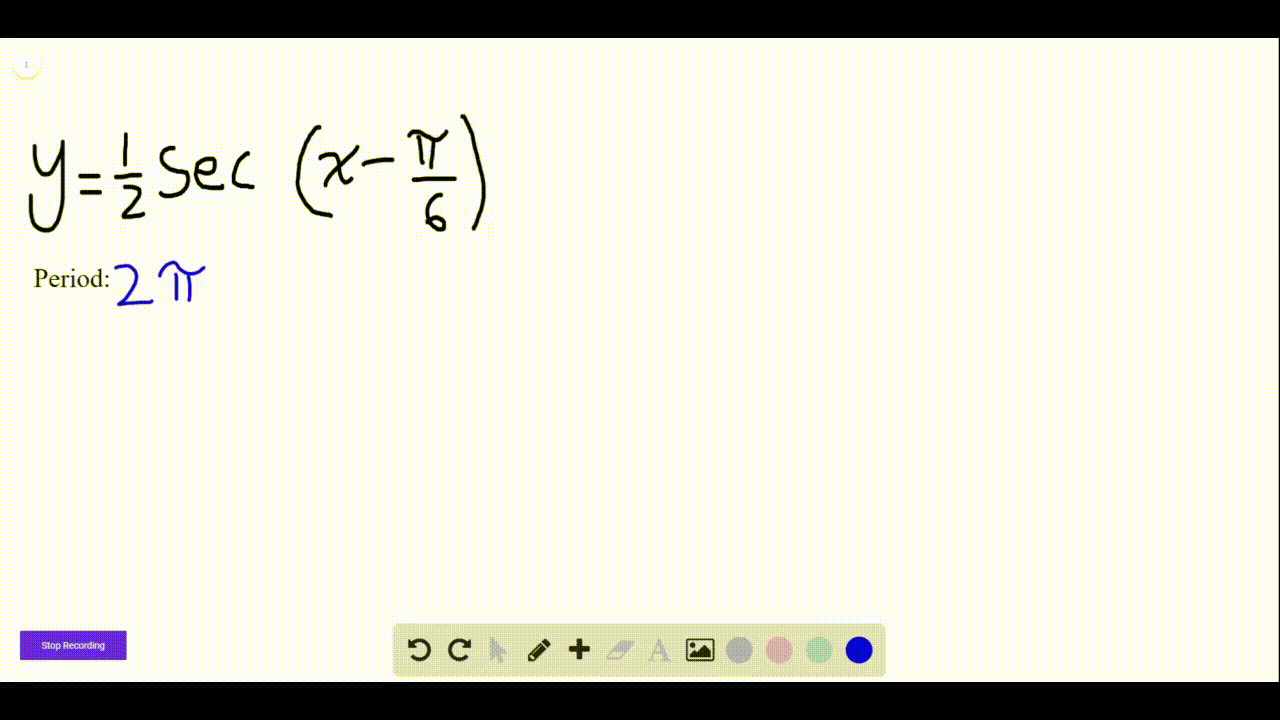Algebra and Trigonometry

$35-60=$ Graphs of Trigonometric Functions with Horizontal
Shifts Find the period, and graph the function.

$$y=\frac{1}{2} \sec \left(x-\frac{\pi}{6}\right)$$

Chapter 6: Trigonometric Functions: Unit Circle Approach
Section 4: More Trigonometric Graphs02:06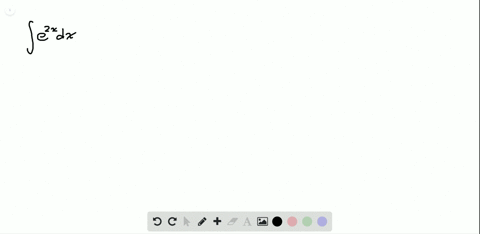Calculus Volume 1

In the following exercises, compute each indefinite integral.
$$\int e^{2 x} d x$$

Chapter 5: Integration
Section 6: Integrals Involving Exponential and Logarithmic Functions00:52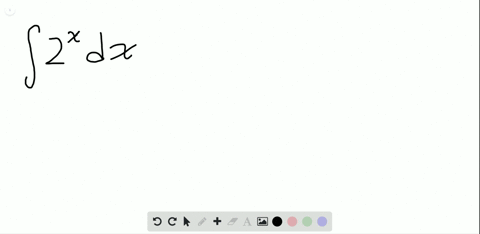Calculus Volume 1

In the following exercises, compute each indefinite integral.
$$\int 2^{x} d x$$

Chapter 5: Integration
Section 6: Integrals Involving Exponential and Logarithmic Functions01:18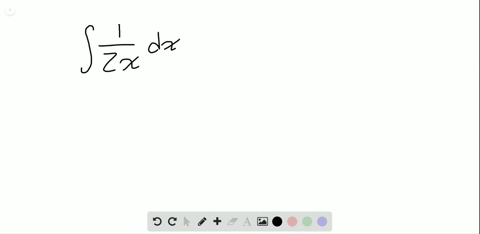Calculus Volume 1

In the following exercises, compute each indefinite integral.
$$\int \frac{1}{2 x} d x$$

Chapter 5: Integration
Section 6: Integrals Involving Exponential and Logarithmic Functions01:41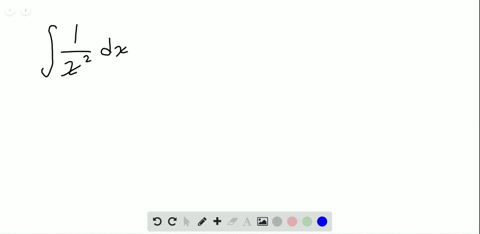Calculus Volume 1

In the following exercises, compute each indefinite integral.
$$\int \frac{1}{x^{2}} d x$$

Chapter 5: Integration
Section 6: Integrals Involving Exponential and Logarithmic Functions01:42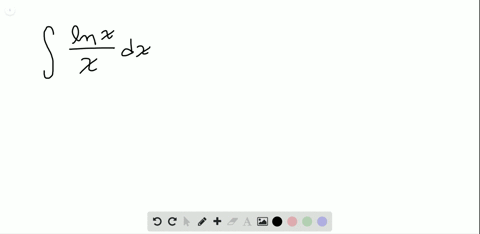Calculus Volume 1

In the following exercises, find each indefinite integral by using appropriate substitutions.

$$\int \frac{\ln x}{x} d x$$

Chapter 5: Integration
Section 6: Integrals Involving Exponential and Logarithmic Functions1 2 3 4 5 ... 62